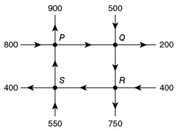## The figure above represents a network of one-way streets.

##### This topic has expert replies
Moderator
Posts: 6595
Joined: 07 Sep 2017
Followed by:20 members

### The figure above represents a network of one-way streets.

by BTGmoderatorDC » Fri Dec 13, 2019 4:56 pmThe figure above represents a network of one-way streets. The arrows indicate the direction of traffic flow, and the numbers indicate the amount of traffic flow into or out of each of the four intersections during a certain hour. During that hour, what was the amount of traffic flow along the street from R to S if the total amount of traffic flow into P was 1,200 ? (Assume that none of the traffic originates or terminates in the network.)

A. 200
B. 250
C. 300
D. 350
E. 400

OA B

Source: Official Guide

Junior | Next Rank: 30 Posts
Posts: 18
Joined: 30 Sep 2019
by henilshaht » Sun Dec 15, 2019 10:50 am
Let's assume the traffic from R to S is x
and traffic from S to P is y.

Now, we know the inward traffic at P is 1200. So,
1200 = 800 + y
y = 400.

At any point, inward traffic will be equal to the outward traffic.
So, using that approach at S, we get the following equation

Inward traffic at S = Outward traffic at S
550 + x = 400 + y
x = 400 + 400 - 550
x = 250

### GMAT/MBA Expert

GMAT Instructor
Posts: 6491
Joined: 25 Apr 2015
Location: Los Angeles, CA
Thanked: 43 times
Followed by:26 members
by [email protected] » Wed Jan 01, 2020 6:43 pm
BTGmoderatorDC wrote:The figure above represents a network of one-way streets. The arrows indicate the direction of traffic flow, and the numbers indicate the amount of traffic flow into or out of each of the four intersections during a certain hour. During that hour, what was the amount of traffic flow along the street from R to S if the total amount of traffic flow into P was 1,200 ? (Assume that none of the traffic originates or terminates in the network.)

A. 200
B. 250
C. 300
D. 350
E. 400

OA B

Source: Official Guide
First let's look at intersection P. Since 1,200 cars flow into P and 900 flow out of P northwardly, 300 must flow out of P eastwardly to Q.

Next, let's look at intersection Q. Since 300 + 500 = 800 cars flow into Q and 200 flow out of Q eastwardly, 600 must flow out of Q southwardly to R.

Lastly, let's look at intersection R. Since 600 + 400 = 1,000 cars flow into R and 750 flow out of R southwardly, 250 must flow out of R westwardly to S.

Alternate Solution:

The total traffic flow into P is the sum of the traffic flow from S to P and the traffic flow into P from east, which is 800. Since the total traffic flow into P is given to be 1,200; the traffic flow from S to P must be 1200 - 800 = 400.

We see that the total traffic flow out of S is 400 + 400 = 800. Since the traffic flow into S from south is 550, the traffic between R and S must be 800 - 550 = 250.

### GMAT/MBA Expert

GMAT Instructor
Posts: 16086
Joined: 08 Dec 2008
Location: Vancouver, BC
Thanked: 5254 times
Followed by:1267 members
GMAT Score:770

### Re: The figure above represents a network of one-way streets.

by [email protected] » Sat Apr 23, 2022 8:30 am
BTGmoderatorDC wrote:
Fri Dec 13, 2019 4:56 pmThe figure above represents a network of one-way streets. The arrows indicate the direction of traffic flow, and the numbers indicate the amount of traffic flow into or out of each of the four intersections during a certain hour. During that hour, what was the amount of traffic flow along the street from R to S if the total amount of traffic flow into P was 1,200 ? (Assume that none of the traffic originates or terminates in the network.)

A. 200
B. 250
C. 300
D. 350
E. 400

OA B

Source: Official Guide
The total amount of traffic flow into P was 1,200
There are 800 cars heading towards P from the left, which means the remaining 400 cars must be heading towards P from below.Since 1200 cars entered intersection P, 1200 cars must leave intersection P.
Since 900 cars are leaving intersection P upwards, the remaining 300 must be leaving intersection P to the right.At this point, we can see that there are 800 cars entering intersection Q, which means there must be 800 cars leaving intersection Q.
Since 200 cars are leaving intersection Q to the right, the remaining 600 cars must be leaving intersection Q downwardsFinally, we can see that there are 1000 cars entering intersection R, which means there must be 1000 cars leaving intersection R.
Since 750 cars are leaving intersection R downwards , the remaining 250 cars must be leaving intersection R to the left,